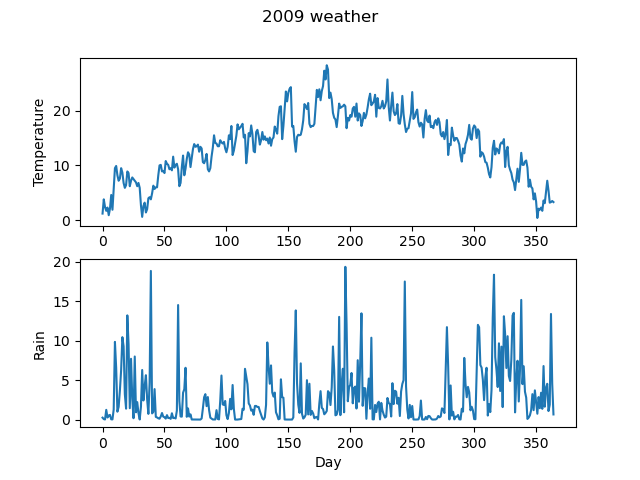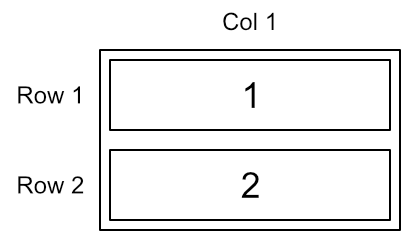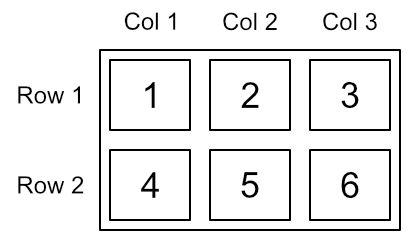# Using subplots in Matplotlib

Martin McBride, 2022-06-25
Tags subplots
Categories matplotlibWe have already seen how to add two data sets to a single line plot or bar chart.

Sometimes it is useful to be able to add several different graphs to the same image. This is done using sub-plots.

## Subplot example

Here are 2 line plots of daily temperature and rainfall for 2009:Here is the code to create this plot:

import matplotlib.pyplot as plt
import csv

with open("2009-temp-daily.csv") as csv_file:
temperature = [x for x in csv_reader]

with open("2009-rain-daily.csv") as csv_file:
rain = [x for x in csv_reader]

days = range(365)

plt.subplot(2, 1, 1)
plt.plot(days, temperature)
plt.ylabel("Temperature")
plt.subplot(2, 1, 2)
plt.plot(days, rain)
plt.xlabel("Day")
plt.ylabel("Rain")
plt.suptitle("2009 weather")
plt.show()


We first read in both data sources, to create the temperature and rain lists, and also the days list as normal.

Subplots are arranged in a grid, like this:This shows that the plots are arranged as 2 rows and 1 column. The plots are numbered 1 and 2.

Here is the code to plot the first graph:

plt.subplot(2, 1, 1)
plt.plot(days, temperature)
plt.ylabel("Temperature")


We create the first subplot using the plt.subplot(rows, cols, index) function. This takes three parameters:

• The number of rows, which is 2 in our case.
• The number of columns, which is 1 in our case.
• The plot number, which is 1 for the first plot. This ensures that the plot is placed in the top position.

We then call the plot and ylabel functions. Because we called the subplot function first, those functions apply to the subplot rather than the whole graph.

We create the second subplot by calling plt.subplot a second time:

plt.subplot(2, 1, 2)
plt.plot(days, rain)
plt.xlabel("Day")
plt.ylabel("Rain")


This time the rows and columns are the same, but we set the plot number to 2 to indicate that this plot should go in the bottom position.

We also add an xlabel set to Day. This is only added to the bottom plot because both plots use the x-axis to show days, so it is not necessary to label both plots.

plt.suptitle("2009 weather")


When we have multiple plots we use suptitle rather than title. This applies a title to the entire image (whereas title can be used to apply a title to each subplot).

## Subplot layout

The case above used 2 subplots, arranged as 2 rows, 1 column. Here is an alternative layout, with 6 subplots arranged as 2 rows of 3 columns:The code to create a plot like this is:

plt.subplot(2, 3, 1)
# create plot 1
plt.subplot(2, 3, 2)
# create plot 2
plt.subplot(2, 3, 3)
# create plot 3
plt.subplot(2, 3, 4)
# create plot 4
plt.subplot(2, 3, 5)
# create plot 5
plt.subplot(2, 3, 6)
# create plot 6


This time each call to subplot has:

• row set to 2.
• cols set to 3.
• index set to the plot number.

Plots are numbered 1 to 6, row by row, as shown in the image above.

We can create more complex layouts, like this:Here, the top row of images is created using a 2 row, 3 column grid, exactly like the previous example. Since these three plots occupy the first three positions, we create them using parameters (2, 3, 1), (2, 3, 2), and (2, 3, 3).

The bottom image is created using a 2 row, 1 column grid, just like the original case from earlier. This time, the image occupies the second position in the grid, so we create it using parameters (2, 1, 2).

If you found this article useful, you might be interested in the course Introduction to Matplotlib by the same author.

#### Popular tags

2d arrays abstract data type alignment and angle animation arc array arrays bar chart bar style behavioural pattern bezier curve built-in function callable object chain circle classes clipping close closure cmyk colour combinations comparison operator comprehension context context manager conversion count creational pattern data science data types decorator design pattern device space dictionary drawing duck typing efficiency ellipse else encryption enumerate fill filter font font style for loop function function composition function plot functools game development generativepy tutorial generator geometry gif global variable gradient greyscale higher order function hsl html image image processing imagesurface immutable object in operator index inner function input installing iter iterable iterator itertools join l system lambda function len lerp line line plot line style linear gradient linspace list list comprehension logical operator lru_cache magic method mandelbrot mandelbrot set map marker style matplotlib monad mutability named parameter numeric python numpy object open operator optimisation optional parameter or pandas partial application path pattern permutations pie chart polygon positional parameter print pure function python standard library radial gradient range recipes rectangle recursion reduce regular polygon repeat rgb rotation roundrect scaling scatter plot scipy sector segment sequence setup shape singleton slice slicing sound spirograph sprite square str stream string stroke structural pattern subpath symmetric encryption template text text metrics tinkerbell fractal transform translation transparency triangle truthy value tuple turtle unpacking user space vectorisation webserver website while loop zip